Skip to main content

# Modeling the internet of things: a hybrid modeling approach using complex networks and agent-based models

## Abstract

Sensors, coupled with transceivers, have quickly evolved from technologies purely confined to laboratory test beds to workable solutions used across the globe. These mobile and connected devices form the nuts and bolts required to fulfill the vision of the so-called internet of things (IoT). This idea has evolved as a result of proliferation of electronic gadgets fitted with sensors and often being uniquely identifiable (possible with technological solutions such as the use of Radio Frequency Identifiers). While there is a growing need for comprehensive modeling paradigms as well as example case studies for the IoT, currently there is no standard methodology available for modeling such real-world complex IoT-based scenarios. Here, using a combination of complex networks-based and agent-based modeling approaches, ​we present a novel approach to modeling the IoT. Specifically, the proposed approach uses the Cognitive Agent-Based Computing (CABC) framework to simulate complex IoT networks. We demonstrate modeling of several standard complex network topologies such as lattice, random, small-world, and scale-free networks. To further demonstrate the effectiveness of the proposed approach, we also present a case study and a novel algorithm for autonomous monitoring of power consumption in networked IoT devices. We also discuss and compare the presented approach with previous approaches to modeling. Extensive simulation experiments using several network configurations demonstrate the effectiveness and viability of the proposed approach.

## Introduction

We live in a time where electronic gadgets and integrated sensors are all around us—from versatile Smartphones and tablets to portable PCs, and from indoor temperature regulators to microwave ovens.  We live in a new world—a world of smart*—where intelligence and connectivity is added to every conceivable object. The vision of the internet of things (IoT) by Ashton (2009) appears to have manifested itself—albeit in unexpected ways. This emergence of the IoT in our everyday lives obviously has numerous implications resulting in a very different environment and society.

Considering that the IoT concept is itself quite new, it is understandable that it is difficult to model. Researchers from the communication systems area often focus primarily on the communication aspects of the IoT and assume that this is essentially the whole thing. That approach however, is problematic—to say the very least. Firstly, considering only the communication aspect of the devices—with little regards to the fact that they are part of our everyday lives—is obviously incorrect. Secondly, while this would have been fine when the technologies were at the stage of proof-of-concept, this is not enough at the current stage when they have even reached commercial proliferation. Additionally, a key problem in modeling the IoT using existing methodologies and tools is that the number of devices in the real world far outnumbers the one used as a proof-of-concept in research papers using traditional simulation tools.

With IoT structures growing at such a fast rate, existing simulation tools such as NS2/3 and others are often unsuitable to effectively model and simulate such systems (Niazi 2008). Agent-based modeling and complex networks (Gershenson and Niazi 2013) can however be considered to model such infrastructures—such as proposed by us previously in cognitive agent-based computing framework (Niazi and Hussain 2013). This framework offers tools and techniques to effectively model different types of complex adaptive systems as well as complex physical systems such as the IoT (Niazi and Hussain 2009).

Previously, to the best of our knowledge, no work has been presented which combines different modeling paradigms from the perspective of complex systems modeling demonstrating how these paradigms may be used to effectively model complex communication networks, in general, and the IoT, in particular.

The novelty of the current paper lies in the usage of agent-based modeling in conjunction with complex networks as part of the cognitive agent-based computing framework—essentially advancing our previous work presented in Laghari and Niazi (2016). The idea is further demonstrated by means of a specific case study modeling complex network topologies for the calculation of power consumption in devices as part of the IoT.

To demonstrate the application of the cognitive agent-based computing framework, we have chosen a particular problem related to estimation of power consumption in networked sensing devices. For numerous reasons, gadgets can tend to act like “invisible” power scattering sources. Persistent power utilization additionally brings about higher air-conditioning bills. The resultant impact on the planet is alarming. This is actually a phenomenon resulting from application of the basic laws of thermodynamics (Dincer 2000).

The key contributions of the current paper are summarized as follows:

We propose a methodology for modeling complex scenarios in the IoT domain using a combination of complex networks and agent-based modeling used in conjunction with each other. We would like to also note our previous introductory work in the domain published in conference proceedings such as (Batool and Niazi 2015; Batool et al. 2014). The current work, however, is both considerably more extensive as well as demonstrates the modeling of IoT dynamics in a number of realistic network topologies—namely small-world and scale-free networks in addition to lattice, and scale-free networks.

The modeling also expands upon insights obtained from our complex network validation methodology presented earlier in (Batool and Niazi 2014).

The structure of the paper is as follows: first, necessary background to understand the methodology is described. This is followed by the model development. Furthermore, results of extensive simulation experiments are presented prior to the conclusion of the paper.

## Background

In this section, a brief background is discussed. First, agent-based modeling paradigm is discussed giving details of why it is well suited for modeling and studying complex consumer networks. Next, self-organization is discussed. This is followed by a brief background of the IoT.

### Agent-based modeling

The idea behind agent-based modeling (ABM) is to create models of a complex system using individuals or agents as the building blocks. This helps in not only simulating systems for design and solution of complex problems but also for the resolution of practical engineering issues (Niazi and Hussain 2011). The simulation is created to implement the operations followed by some defined rules where components interact with each other to simulate complex environments and even predict emergent behavior (Niazi 2013). This is done to develop an understanding of the complex natural environment with advanced simulation techniques (Bonabeau 2002). ABM models are commonly actualized utilizing PC reproductions either by some custom programming or particularly created ABM toolkits taking into account a more profound comprehension of the conduct of the general framework in light of the individual operators (Gershenson and Niazi 2013). To model such large complex networks agent based modeling and simulation tools are therefore a natural choice (Niazi and Hussain 2011). In previous work such as (Niazi and Hussain 2009) it has been demonstrated how complex communication networks involving autonomous and interacting agents can be modeled using these agent-based modeling tools.

### Self-organization

Self-organization is the ability of a system to organize in a meaningful structure without any external force as long as certain rules are enforced in the system (Ashby 1991). This ability exits in many natural systems such as animals (Hemelrijk and Hildenbrandt 2012), human cells (Kadoshima et al. 2013), galaxies (Cen 2014) and other processes (Nicolis and Prigogine 1977) such as crystallization. Self-organization emerges as a means of maintaining the equilibrium in a system especially where the agents are interdependent.

Self-organization is considered to be an important ability in networks for successful networking (Barabási and Albert 1999). This is because the behavior is not controlled by external systems and the effect is on whole system. This organization is robust and gives the systems a capability to sustain and self-repair the damages or problems occurring in a system. Complex network such as Small-world and Scale-free networks also exhibit self-organizing capabilities (Wang and Chen 2003; Siemens 2005). Their organizational structure is discussed in Section V and the importance of such networks is discussed next. An alternate approach to modeling complex phenomena is using system dynamics (Azar 2012). Additionally, soft computing also offers techniques not only to model but also control complex systems (Zhu and Azar 2015).

### Complex networks

One way of modeling complex adaptive systems and complex physical systems is by using complex networks (Niazi and Hussain 2013). Complex networks are loaded graphs ranging from simple networks such as lattice to random networks. These network types are important building blocks of complex systems however neither of them might behave similar to real-world empirical networks in terms of their topological structure (Newman 2010). In the real world, networks need to be analyzed to understand the exact features. Two typical network models, which have been categorized in literature in terms of corresponding to real-world networks, are the so-called small-world and the scale-free networks.

For this paper, the following network models have been implemented using ABM:

1. 1.

Small-world network

2. 2.

Scale-free network

3. 3.

Random network

4. 4.

Lattice network

Following is a brief introduction of these networks:

#### Small-world network

An associated network with a high chart distance across and when edges are included haphazardly, the measurement diminishes radically. This network is sometimes also called a “six degrees of separation” in social networks where any individual turns to be linked with any other individual by maximum of six links. In this network, the nodes mostly are not neighbors to each other; instead, they become neighbors by making links with other nodes also. Therefore, in simple words it can be understood as fewer nodes with more links. Mathematically, it is satisfied by:

$$L\alpha Log(N)$$
(1)

A small-world system has the accompanying properties:

1. 1.

Clustering coefficient: it is a measure of degree to which hubs in a chart tend to bunch together. Clustering coefficient for one node is calculated by following formula:

$$Ci = \frac{number\,of\,links}{Maximum\,Number\,of\,Links}$$
(2)

Clustering coefficient of entire system is ascertained by following formula:

\begin{aligned} C = \frac{1}{N}\left(\sum\limits_{i} {Ci}\right) \hfill \\ \hfill \\ \end{aligned}
(3)

N = Number of nodes, C = Clustering coefficient for each node i.

2. 2.

Mean-shortest path length: The small-world components has been perceived in numerous real world graphs, for example, social networks, neural systems, programming frameworks, road plans, food chains and electric power matrices and so on. Small-world systems are made of connections of a d dimensional grid where we supplant a fraction P with connections, arbitrarily, by interjecting it among two instances of a usual lattice i.e. (P = 0) and a random graph (P = 1). Considering a ring having n vertices, among which each is associated with its k closest neighbors by undirected edges. At that point, a vertex and edge is chosen for connecting with nearest neighbor in a clockwise direction. Edge is reconnected to a vertex picked consistently at irregular over the whole ring on premise of probability p, by maintaining a strategic distance from the duplicate edges or leaving the edge set up. This procedure is rehashed on moving clockwise around the ring and considering every vertex until one lap finishes. Next, edges are viewed as the vertices connected to their second-closest neighbors clockwise utilizing the same process as outline above. This continues until every edge in the original lattice has been considered at least once.

#### Scale-free network

Scale-free network is one of the complex networks in which the connectivity of nodes with the other nodes is extremely uneven. This means that there are a few nodes that have dense connectivity, while majority of the nodes have a comparatively less number of connections. Densely connected nodes are often termed as the “Hubs”. Scale-free network is considerably focused on these nodes. Modeling the dynamics in such systems plainly exhibits that a scale-free network can develop when systems increment by adding hubs to an officially existing system, and those hubs have a tendency to join specially to the hubs which are as of now very much associated. The probability that a hub will interface with k different hubs in a given system is specifically corresponding to $$k^{- \gamma}$$, which suggests that a scale-free network takes after a Power Law of degree distribution of hubs.

To begin the network with an underlying condition of $$m_{o}$$ hubs ($$m_{o}$$ ≥ 2) the degree of every hub ought to be at least 1, in light of the fact that generally hubs will stay disengaged from whatever is left of the system. New hubs in a steady progression are included. The new node is connected to the old node, which has the high probability of number of links already existing. Formally, the probability $$p_i$$ that the new hub is associated with hub i is

$$\prod {(k_{i})} = \frac{{k_{i}}}{{\sum\nolimits_{j} {k_{j}}}}$$
(4)

In Eq. 4, $$k_{i}$$ is the degree of hub i in real networks is with the end goal that, there is a nonzero likelihood that another hub will join to a confined hub display in the network. Heavily linked nodes called ‘Hubs are more probable and rapidly tend to accumulate much more connections, while hubs with just a couple connections are probably not going to be picked as the goal for another connection. It implies that the new hubs will have an inclination to get associated themselves to the effectively existing substantial connected hubs. The figures below, shows how this method of how the nodes choose to attachment themselves to make a scale free network. According to scale-free model, this one at a time attaching of node helps the network to grow. Then the new node in the network tend to prefer to get connected to the nodes with heavy links, meaning that it tries to attach the node near itself which has the highest degree.

#### Random network

Consider a network framed by interfacing diverse vertices in an arbitrary way, scientifically alluded to as the Erdős–Rényi random network model (ERDdS and Wi 1959). Formally, a random network $$G_R(n,p)$$ is framed with edges associated with a likelihood, $$p$$ given that 0 < $$p$$ < 1.

A Random Network is shaped by associating vertices with each other in an arbitrary way. The connectivity of nodes is not dependent on the links of the nodes. For analyzing a random network, take a simple example where edges are added at random to the n isolated vertices of a graph, where n is fixed. One way to understand this is to assume that for all $$nC_{2} = \frac{n(n - 1)}{2}$$ edges that are viable in a graph with n vertices, there are equal chances that a particular edge will be separately added to the graph. One way is to simply add the edges at random. This is similar to tossing a coin in the air. A sub graph is created for the edge when the coin comes up heads for a particular link.

Specifically, random graphs do not provide solution to tackle with such networks, which grow with time hence additional ideas are needed to bring them to practicality, for which the complex networks have been evolved.

#### Lattice network

A lattice network $$G_L$$ is made out of vertices with the end goal that every vertex associates with four different vertices to frame a work as a four-connected grid. This network structure has a growing nature and thus allows unlimited capacity which increases the functionality and simplifies routing in network (Beshai 2005). The edge modules are addressed using logical coordinates, one coordinate being assigned for each of the N dimensions. This simplifies routing and permits each edge module to compute its own routing tables (Beshai 2005).

### Internet of things

The IoT is a rapidly growing infrastructure and concept, primarily due to standard connectivity features in consumer electronics devices (Ashton 2009). IoT has resulted in consumer networks with remote device connectivity. Fully integrating IoT with technology will give huge opportunities for information and communication technologies (ICT) sector, which will give rise to the development of new applications, software and devices. As this area has not fully developed and needs more researches, this area is still lacking a standard architecture, which can be followed for further development and advancements. Many challenges have been recognized which hinder the deployment of IoT. Few of the challenges are the interoperability issue of devices while providing security, privacy and functionality and should have low computational power and energy capacity as it is a fundamental requirement for the upcoming solutions (Ashton 2009).

## Model development for self-organized power consumption approximation algorithm

Here, we propose a model on self-organized power consumption approximation (SOPCA) algorithm in the IoT. Moreover, a brief analysis is given on the simulation environment and model development of the networks.

### Definition 1 Scenario of the IoT

To sensibly show the IoT, a huge set S of organized buyer electronic gadgets associated with each other in various setups is considered. To show distinctive potential outcomes of availability for these gadgets, two diverse network modeling strategies—random network connectivity strategy ($$C_R$$) providing irregular system availability procedure and lattice network connectivity strategy ($$C_L$$) providing cross section arrange network methodology are illustrated. Next, the proposed algorithm on two more network strategies small-world, ($$C_S$$) and scale-free, ($$C_{Sf}$$) connectivity strategies is implemented. Practical implementations can result any multi-agent programming toolbox equipped for execution on these consumer devices (Ughetti et al. 2008). An abstract overview of concept illustration of implementing the proposed algorithm on mentioned networks is shown in Fig. 1.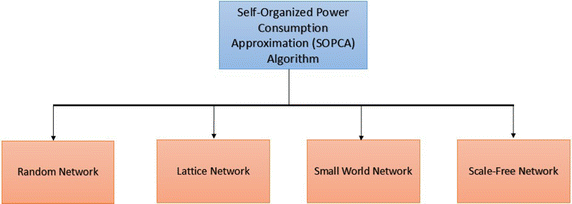Fig. 1

### SOPCA algorithm

The main concept of SOPCA algorithm is presented in  Fig. 2, earlier proposed in (Batool and Niazi 2015). The idea is to exploit wireless connectivity between peer devices in conjunction with a server. Devices are able to locate and communicate with other devices by means of an agent—termed as the energy sniffer agent (ESA). ESAs can find other devices in addition to keeping an estimate of the energy consumption discovered while exploration. Each ESA has a time-to-live (TTL), which when completed can either result in their termination or else have them return to the sink nodes. It is noted here that comparable thoughts of availability of different customer gadgets have additionally already been presented in (Niazi 2008; Niazi and Hussain 2011). Source nodes can find different nodes in their nearness by utilizing either signal strength or global positioning system (GPS). ESAs overhaul their inner factors in light of perception of nearby energy utilization and next proceed onward to different nodes. To stay away from re-routing through various discovered gadgets, ESA’s can likewise set a flag on each device.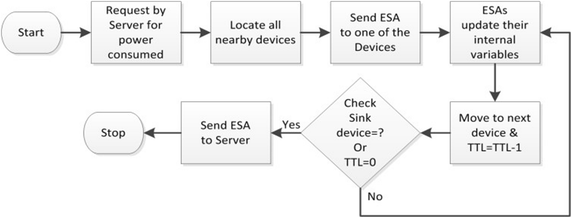Fig. 2

Akyldiz noticed that energy cost of transmitting 1 KB at a separation of 100 m is around the same as that for executing 3 million instructions by a 100 million instructions for each second(MIPS)/W processor (Akyildiz et al. 2002). In other words, transmission of the packets and gathering expends a similar measure of energy cost for short-range correspondence. This power is not only used for transmitting and receiving data but also for turning the devices on and off. Even though this start-up time (turning on and off) is very small (100 μs), still this time is noticeable. Radio power consumption (Pc) is presented as:

$$P_c = N_T[P_T(T_{on} + T_{st}) + P_{out}(T_{on})] + N_R[P_R(R_{on} + R_{st})]$$
(5)

where $$P_c$$: power consumption, P out : output power of the transmitter, $$P_R$$: power consumed by the receiver, $$N_T$$: number of times the transmitter is exchanged on per unit time, $$N_R$$: number of times the receiver is exchanged on per unit time, $$T_{on}$$: transmitter on time, $$T_{st}$$: transmitter startup time, $$R_{on}$$: receiver on time, $$R_{st}$$: receiver startup time.

### Model development of networks

Here, four different networks are modeled. Two of the networks belong to non-real world and two are from real-world: random and lattice, small-world and scale-free networks. Next, these network models are described.

#### Random network

Figure 3 shows the simulation algorithm for creating random network. For all the nodes, shape and color are set. These nodes are placed randomly on the grid. At the point when the nodes have been made, every node joins with a haphazardly picked node however never to itself. Here, a probability, p for creating links within network is set. According to appropriate p, the links will neither be loosely connected nor have too congested connections in networks. And lastly, a source node randomly is chosen.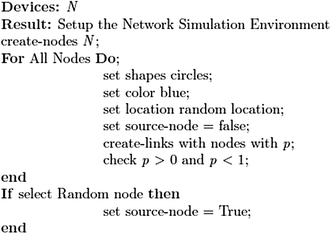Fig. 3

#### Lattice network

In the procedure directed in Fig.  4, each patch grows one node on the network. Source nodes are to be chosen arbitrarily after the patch area is filled with nodes.Fig. 4

#### Small-world network

In Fig.  5, the simulation algorithm for creating small-world network is described. Initially nodes are created and shape is set. Any success variable is set as false. While in case of an unsuccessful attempt, perform wire-them function and in case of success perform do-calculations function. Any variable named clustering-coefficient value is set as clustering-coefficient; shortest-path-length-of-lattice is set as a shortest-path. If there are no-nodes left, stop if there are nodes then link is created with other nodes but if count links are greater than number of links than it will make the link die.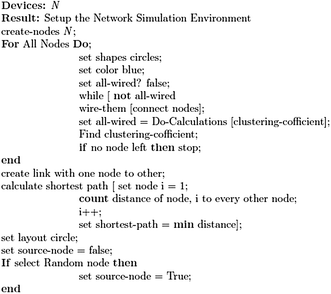Fig. 5

In order to find the clustering coefficient a function is made for it. The clustering-coefficient and total is set initially as 0.

Next, the nodes interface with its neighbors is checked if: link-neighbors are not exactly or equivalent to one then the node-clustering-coefficient is set unspecified. Nodes with link-neighbors greater than 1 will play out the calculations for finding the clustering-coefficient. Clustering-coefficient is calculated at all nodes. It is summed and stored it altogether. Lastly, minimum separation is taken.

#### Scale-free network

To make a scale-free network, the simulation algorithm as in Fig.  6 is described. The ‘node = nobody’ makes a node in the network which do not connect to any other node as it is the main node in the system. Function ‘make node 1’ makes another node in the system which connections to node 0 as the function ‘old-node! = nobody’ turned true, in the Fig.  6. At the point when the initial two nodes have been made and connected together in the network, function ‘add node’ keeps running until the number of nodes is less than the number-of-nodes, N.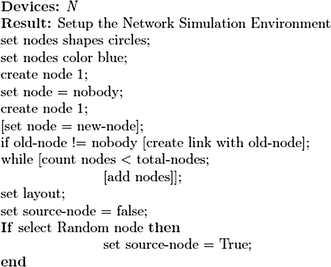Fig. 6

### Simulation setup

To demonstrate the utility of the proposed algorithm, it is implemented on various network topologies with different configurations discussed later. For comparison, random as well as real world networks are considered. Therefore, before modeling the complex system as more realistic networks, some fundamental principles need to be defined.

In order to test the models and SOPCA algorithm on any of the simulator, some physically meaningful and appropriate metrics to characterize both the simulated and actual event lists are identified.

An energy model is utilized to evaluate the power consumption. A basic model expected by Lin et al. (2012) have the radio dissipation $$E_{elec} = 50\,{\text{nJ/bit}}$$ to run the transmitter or receiver hardware $$E_{amp} = 0.1\;{\text{nJ}}/{\text{bit}}/{\text{m}}^{2}$$ and for the transmission amplifier to accomplish an adequate $$E_{b}/N_{o}$$. In this way, to transmit a k-bit message a separation d utilizing this radio model, the radio exhausts:

$$E_{Tx} (k,d) = E_{elec} k + \varepsilon_{amp} kd^{2}$$
(6)

While to get this message, the radio uses:

$$E_{Rx} (k) = E_{elec} k$$
(7)

Putting values in Eq. (6), where range, R = 10; $$\varepsilon_{amp} = 0.1\,{\text{nJ/bit/}}\text{m}^{2}$$, $$E_{elec} = 50\, {\text{nJ/bit}}$$

K = message = 64 bytes = 64.8 = 512 bits

\begin{aligned} E_{Tx}(k,d) &= \left({50\cdot 10^{- 9}} \right)\cdot 512 + \left({0.1 \cdot 10^{- 9}} \right)\cdot 512 \cdot \left({10} \right)^{2} \\ &= \left({2.56 \cdot 10^{- 5}} \right) + \left({5.12 \cdot 10^{- 6}} \right) \\ &= \left({3.072\cdot 10^{- 5}} \right)\\ E_{Tx}(k,d) &= 30.72\,\upmu {\text{J}} \end{aligned}

Next, values are calculated using Eq. (7) in order to estimate the received energy. Where R = 10:

\begin{aligned} E_{Rx}(k) &= E_{elec}k\\ &= \left({50 \cdot 10^{- 9}} \right)\cdot 512 \\ &= \left({2.56 \cdot 10^{- 5}} \right) \\ E_{Rx}(k) &= 25.6\,\upmu {\text{J}} \end{aligned}

Finally, total energy is obtained by adding transmit and receive energies.

For R = 10:

\begin{aligned} E &= E_{Tx}(k,d) + E_{Rx}(k) \hfill \\ E &= 30.72 \,\upmu {\text{J}} + 25.6\,\upmu {\text{J}} \\ E &= 56.3 \, \upmu {\text{J}} \end{aligned}

### Experiments and metrics

To see the behavior of how much energy is consumed by a message when going from the source node to the sink node utilizing different path every time. Therefore, parameters are swept and observed for which combination it dissipates what energy. For the models, nodes are deployed according to their network configurations. The white nodes are the source nodes, which will generate messages and transfer it to the nearest possible node in its transmission range. This process of transferring of message to the node keeps on going until the message reaches to the sink node, which is red in color, or the time-to-live (ttl) of message expires. As the message moves to the nearest possible node, the transmitter node’s energy decreases. Nodes initial energy is set to 0.25 J. When a node transmits the message, energy of 0.015 J is consumed by approximately (for a message containing 64 bytes of data). The simulated test environment is set according to the following settings:

• 100 m × 100 m2 region

• Number of nodes = 300, 400, 500

• Number of sources = 5, 10, 15, 20

• Transmission range = 10 m

• Networks: random network, lattice network, small world network, scale-free network

• No. of runs = 4 (1, 10, 20, 30 runs)

## Results and discussion

### Estimation of energy consumption

Here, we examine energy consumption estimation. The idea of energy consumption includes three separate major tasks—namely sensing, computation, and communication. Previous work has noted that communication is one of the most power-consuming task amongst these three (Kimura and Latifi 2005). The paper estimates an almost equal transmission and reception cost for short-range communication. Therefore, a realistic model can initialize devices with energy of 0.25 J. Besides, a transmission energy of 0.015 J may be used for messages limited to 64 bytes (Lin et al. 2012). These basic parameters are suggested to be kept for a realistic estimation of power consumption in networks.

### Discussion of simulation results

The proposed mechanism is simulated and shows that it can measure the energy released by the electronic devices connected over a network irrespective of the topology it follows. The experiments were averaged over 30 executions. These experiments were performed for N = 300, 400 and 500 for varying number of source nodes. Total energy consumption was measured in mili Joules (mJ), simulated in a transmission range set to R = 10 m. Also see Table 1 for simulation parameters used and Table 2 for estimation power consumption for networks consisting of N = 500.

Networks are simulated with different configurations using ABM tool and implemented SOPCA algorithm. The algorithm was tested to analyze the power consumption effects on smaller and larger networks.

For random network, Figs.  7, 8 and 9 show the networks for varying number of nodes. They show the power consumption increases as the networks become larger and also when network is tested for increasing number of runs.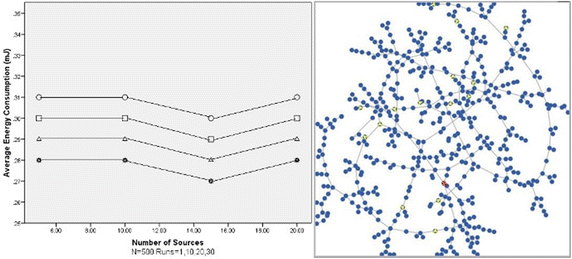Fig. 7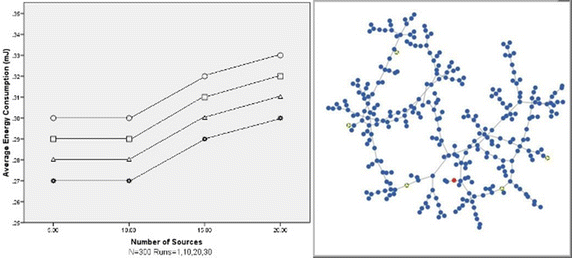Fig. 8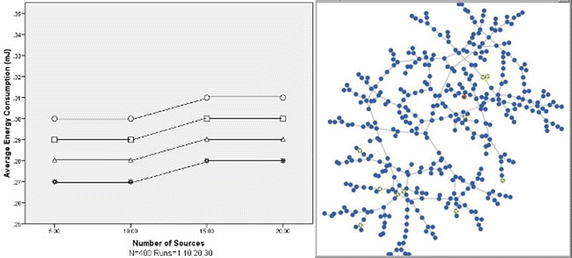Fig. 9

For lattice network, Figs.  10, 11 and 12 show the networks for varying number of nodes. The figures show power consumption behavior as random for different runs but it remains the same for different sizes of networks. It is because the topology has been remained the same throughout.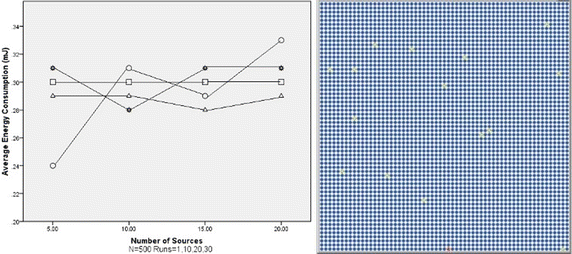Fig. 10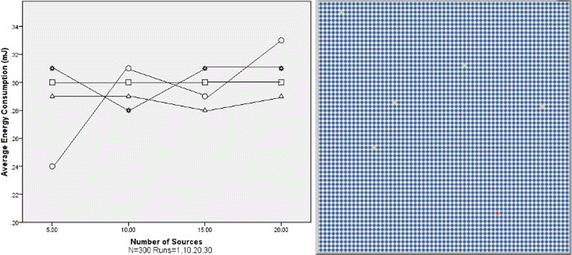Fig. 11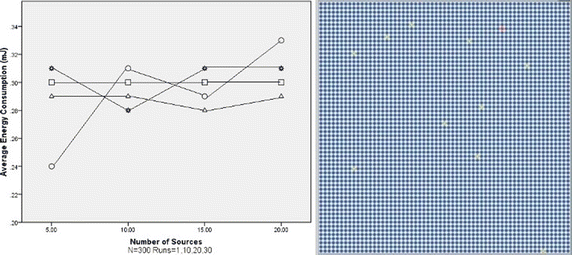Fig. 12

For small-world network, Figs.  13, 14 and 15 show the networks for varying number of nodes. The figures show power consumption behavior is almost similar with different number of network sizes but slightly different for varying number of source nodes. This is due to the structure of the network.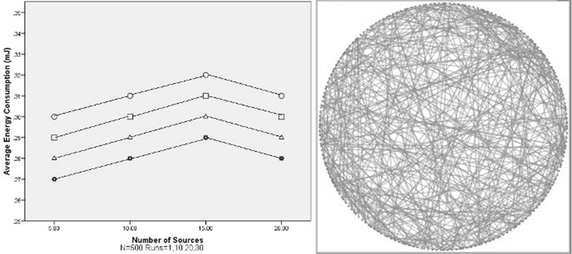Fig. 13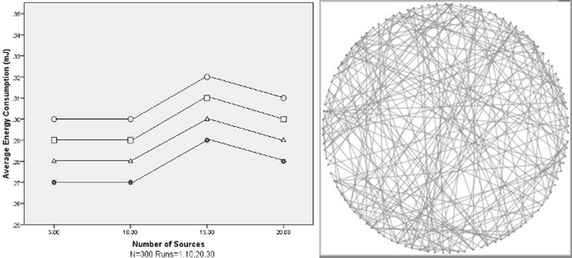Fig. 14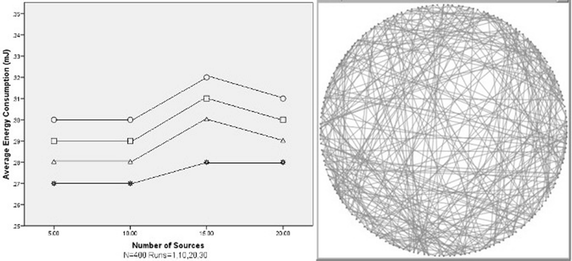Fig. 15

For scale-free network, Figs.  16, 17 and 18 show the networks for varying number of nodes. The figures show power consumption behavior is almost similar with different number of network sizes but slightly different for varying number of source nodes. Similarly, this is also due to the network topology.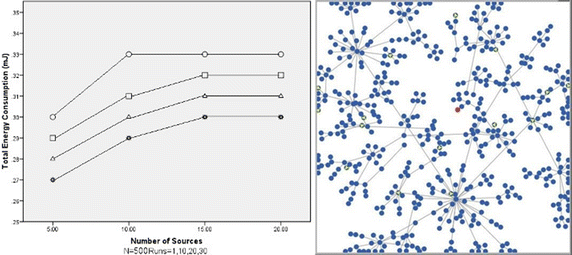Fig. 16Fig. 17Fig. 18

### Related work

Some of the key recent papers in modeling the IoT include paper by Laghari and Niazi (2016) which apply the cognitive agent-based computing to model power consumption architecture. However, their paper does not take care of large-scale complex networks such as in the domain of random/lattice, scale-free and small-world networks.

In like manner, the paper by Altamimi and Ramadan (2016) presents a way to deal with displaying IoT using a gateway approach. Their proposed approach focuses on the use of gateways for more effective communication. Likewise Mashal et al. (2016) present the use of graph-based approaches in recommendation systems for the IoT. Zhang et al. (2016) demonstrate the use of Petri nets to model interactions between the sensors and the environment.

## Conclusion

In this paper, we show for the first time, a way to deal with displaying the IoT by consolidating agent-based modeling with complex networks utilizing methods exhibited before under the cognitive agent-based computing framework. To show the proposed demonstrating system, a self-organizing distributed algorithm for dynamic approximation of power utilization in organized customer electronic gadgets is additionally exhibited. As an approval of ideas, extensive simulation experiments have been exhibited. SOPCA algorithm was tested over random, lattice, small-world and scale-free networks. These networks are an estimate of exceptionally dense networks of consumer electronic gadgets, for example, internet of things. The newness of the exhibited work lies both in the modeling of the Internet of Things utilizing complex networks and in the utilization of agent-based models in addition to the proposed SOPCA algorithm. The essential thoughts from SOPCA algorithm can be further investigated by the assessment of flooding over these systems. The SOPCA algorithm has been implemented in realistic standard complex network topologies. Further analysis was conducted to measure energy consumption by nodes using varying metrics. In addition, we have demonstrated how the total network power consumption of SOPCA algorithm in the IoT networks can be evaluated by means of varying different metrics. In future, this work can be extended by implementing in IoT real network problem. Not only may this; to further elaborate our research work contributions, flexible simulation parameters for network strategies be used.

## References

1. Akyildiz IF, Su W, Sankarasubramaniam Y, Cayirci E (2002) Wireless sensor networks: a survey. Comput Netw 38(4):393–422

2. Altamimi AB, Ramadan RA (2016) Towards internet of things modeling: a gateway approach. Complex Adapt Syst Model 4(1):25. doi:10.1186/s40294-016-0038-3

3. Ashby, W. R. (1991) Principles of the self-organizing system. In: Ashby WS (ed) Facets of Systems Science. Springer, US, pp 521–536

4. Ashton K (2009) That ‘internet of things’ thing. RFiD J 22(7):97–114

5. Azar AT (2012) System dynamics as a useful technique for complex systems. Int J Ind Syst Eng 10(4):377–410

6. Barabási A-L, Albert R (1999) Emergence of scaling in random networks. Science 286(5439):509–512

7. Batool K, Niazi MA (2014) Towards a methodology for validation of centrality measures in complex networks. PLoS ONE 9(4):e90283

8. Batool K, Niazi MA (2015) Self-organized power consumption approximation in the internet of things. In: 2015 IEEE international conference on consumer electronics (ICCE). IEEE, LasVegas, USA, pp 313–314

9. Batool K, Niazi MA, Sadik S, Shakil ARR (2014) Towards modeling complex wireless sensor networks using agents and networks: a systematic approach. In: 2014 IEEE region 10 conference TENCON 2014, 22–25 Oct 2014. pp 1–6. doi:10.1109/TENCON.2014.7022378

10. Beshai ME (2005) Multi-dimensional lattice network. Google Patents

11. Bonabeau E (2002) Agent-based modeling: methods and techniques for simulating human systems. Proc Natl Acad Sci 99(suppl 3):7280–7287

12. Cen R (2014) Temporal self-organization in galaxy formation. Astrophys J Lett 785(2):L21

13. Dincer I (2000) Thermodynamics, exergy and environmental impact. Energy Sources 22(8):723–732

14. ERDdS P, Wi A (1959) On random graphs I. Publ Math Debrecen 6:290–297

15. Gershenson C, Niazi MA (2013) Multidisciplinary applications of complex networks modeling, simulation, visualization, and analysis. Complex Adapt Syst Model 1(1):17

16. Hemelrijk CK, Hildenbrandt H (2012) Schools of fish and flocks of birds: their shape and internal structure by self-organization. Interface Focus 2(6):726–737

17. Kadoshima T, Sakaguchi H, Nakano T, Soen M, Ando S, Eiraku M, Sasai Y (2013) Self-organization of axial polarity, inside-out layer pattern, and species-specific progenitor dynamics in human ES cell—derived neocortex. Proc Natl Acad Sci 110(50):20284–20289

18. Kimura N, Latifi S (2005) A survey on data compression in wireless sensor networks. In: International conference on information technology: coding and computing, 2005. ITCC 2005. IEEE, New York, pp 8–13

19. Laghari S, Niazi MA (2016a) Modeling the internet of things, self-organizing and other complex adaptive communication networks: a cognitive agent-based computing approach. PLoS ONE 11(1):e0146760

20. Laghari S, Niazi MA (2016b) Data from: modeling the internet of things, self-organizing and other complex adaptive communication networks: a cognitive agent-based computing approach. Dryad Digial Repos. doi:10.5061/dryad.mq793

21. Lin C, Wu G, Xia F, Li M, Yao L, Pei Z (2012) Energy efficient ant colony algorithms for data aggregation in wireless sensor networks. J Comput Syst Sci 78(6):1686–1702

22. Mashal I, Alsaryrah O, Chung T-Y (2016) Testing and evaluating recommendation algorithms in internet of things. J Ambient Intell Humaniz Comput 7(6):889–900. doi:10.1007/s12652-016-0357-4

23. Newman M (2010) Networks: an introduction. Oxford University Press, Oxford

24. Niazi MA (2008) Self-organized customized content delivery architecture for ambient assisted environments. Paper presented at the Proceedings of the third international workshop on use of P2P, grid and agents for the development of content networks, Boston

25. Niazi MA (2008) Self-organized customized content delivery architecture for ambient assisted environments. In: Proceedings of the third international workshop on use of P2P, grid and agents for the development of content networks. ACM, New York, pp 45–54

26. Niazi MA (2013) Complex adaptive systems modeling: a multidisciplinary roadmap. Complex Adapt Syst Model 1(1):1

27. Niazi M, Hussain A (2009) Agent based tools for modeling and simulation of self-organization in peer-to-peer, ad-hoc and other complex networks. IEEE Commun Mag 47(3):163–173

28. Niazi M, Hussain A (2011a) Agent-based computing from multi-agent systems to agent-based models: a visual survey. Scientometrics 89(2):479–499. doi:10.1007/s11192-011-0468-9

29. Niazi MA, Hussain A (2011b) A novel agent-based simulation framework for sensing in complex adaptive environments. Sens J IEEE 11(2):404–412

30. Niazi MA, Hussain A (2011) Enhancing the shopping experience: Bluetooth-based cooperative deal shopping. IEEE Pervasive Comput 10(2):44–47

31. Niazi MA, Hussain A (2013) Cognitive agent-based computing-I: a unified framework for modeling complex adaptive systems using agent-based & complex network-based methods. Springer Briefs in Cognitive Computation, Dordrecht. doi:10.1007/978-94-007-3852-2

32. Nicolis G, Prigogine I (1977) Self-organization in nonequilibrium systems, vol 191977. Wiley, New York

33. Siemens G (2005) Connectivism: a learning theory for the digital age. Int J Instr Technol Distance Learn 2(1):3–10

34. Ughetti M, Trucco T, Gotta D (2008) Development of agent-based, peer-to-peer mobile applications on ANDROID with JADE. In: The second international conference on mobile ubiquitous computing, systems, services and technologies, 2008. UBICOMM’08. IEEE, New York, pp 287–294

35. Wang XF, Chen G (2003) Complex networks: small-world, scale-free and beyond. Circuits Syst Mag IEEE 3(1):6–20

36. Zhang F, Xu Y, Chou J (2016) A novel petri nets-based modeling method for the interaction between the sensor and the geographic environment in emerging sensor networks. Sensors 16(10):1571

37. Zhu Q, Azar AT (2015) Complex system modelling and control through intelligent soft computations. Springer, Berlin

Download references

## Authors’ contributions

Overall, the authors contributed equally to the manuscript. Specifically, MN conceived the idea for the paper. MN and KB developed the simulation models. KB executed the simulation experiments. Both authors analyzed the simulations. Both authors wrote the paper. Both authors read and approved the final manuscript.

### Competing interests

The authors declare that they have no competing interests.

### Funding

The authors received no specific funding for the manuscript.

## Author information

Authors

### Corresponding author

Correspondence to Muaz A. Niazi.

## Rights and permissions

Open Access This article is distributed under the terms of the Creative Commons Attribution 4.0 International License (http://creativecommons.org/licenses/by/4.0/), which permits unrestricted use, distribution, and reproduction in any medium, provided you give appropriate credit to the original author(s) and the source, provide a link to the Creative Commons license, and indicate if changes were made.

Reprints and Permissions